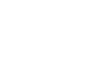How many minutes in a year? - letsdiskussTrishna Dhanda

Self-Starter!!!!! | Posted on | others

How many minutes in a year?

19
0

Student | Posted on

A year consists of 365 days or you can say 52 weeks. And also a year consists of 525600 minutes. Time is a never-ending river of human life. If you respect your time and use it accordingly to your best things. Then every moment of your life will be a good thing. But if you don't respect the time and just live in your comfort zone.Your karma will show you the effect of time. Wake and run for your goals because the world is a great Gym for winners and a drastic place for losers.

27
0

Writer | Posted on

Have you ever thought about how many minutes are there in a year? Or do you have an interest in knowing about minutes in a year? Now you must be feeling that you have missed something interesting in life i.e. interesting calculation of minutes in a year. Well, it is equally important to calculate minutes in a week, and minutes in a day as well. Do not worry this answer will help you to remain updated about the process of calculating minutes in a year.

Firstly, let us know the minutes in a year in numbers: There are 525600 minutes in a year. Great. Right! It is far more interesting to follow the calculation process of minutes in a year than only to know the figure.

How to calculate how many minutes in a year?

Now let us dive into the journey of calculating minutes in a year. Before that, we need to know how many hours per day in a year. Once you are done with the calculation of the number of hours, multiply it by 60. And the result we get is known as the number of minutes in each hour. Its practicality is not as hard as it feels. Look carefully at the calculation of the number of hours per year for better experience:

Wait, remember you need to know the calculation of minutes per day. Because you need the time for the calculation of hours per year. Once you are confirmed about the minutes per day, multiply the result by days in a normal year i.e. 365 days. It goes like 24×368=8760 hours per year. We know that one hour is equivalent to 60 minutes. You need to multiply hours per year by 60 i.e. 8760×60=525600 minutes per year.Image Source: Google

Remember there are not 365 days in a year. Some years have 365 and a quarter. One quarter is equivalent to 6 hours. So in the following cases, the calculation goes like 24×365×60 minutes x 60 hours i.e. 8766 hours per year. So now we are ready with hours each year.

We have reached our final destination in a year is 8766×60= 525960 minutes in a year if we consider 365 and a quarter day in a year.

We all are well aware that a leap year always occurs after 4 years and the year consists of 366 days. And remember the minutes in a normal year is always less than that of a leap year. If we consider a leap year, the calculation goes like 366 days x 24 hours x 6 minutes that is equivalent to 525600 minutes in a year(leap).Image Source: Google

This was an overall calculation process for finding out how many minutes are in a year. How about calculation minutes in a week along with the calculation of minutes in a year. Well, its calculation needs conversion of minutes into a week. And the calculation goes like division of the time by the conversion ratio. And remember there are 10080 minutes in a week.

You must also know the conversion of years to hours as this calculation is frequently utilized in several cases.

The conversion table related to years to hours:
Year [y] Hour [h]
0.01 y 87.6 h
0.1 y 876 h
1 y 8760 h
2 y 17520 h
3 y 26280 h
5 y 43800 h
10 y 87600 h
20 y 175200 h
50 y 438000 h
100 y 876000 h
1000 y 8760000 h

Here comes the conversion of years to hours:
1 y = 8760 h
1 h = 0.0001141553 y

Say for example: convert 15 y to h:
15 y = 15 × 8760 h = 131400 h

So we have tried to give a clear idea of calculation about how many minutes are there in a year and overall knowledge of the calculation process of minutes in a week. You have also come across the process of converting years to hours.

0
0